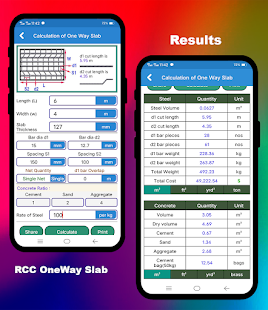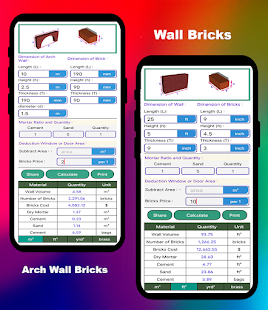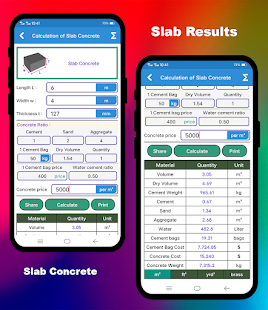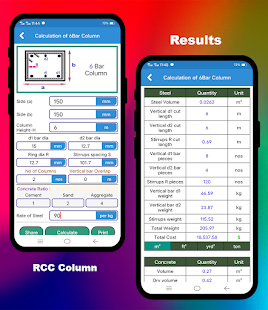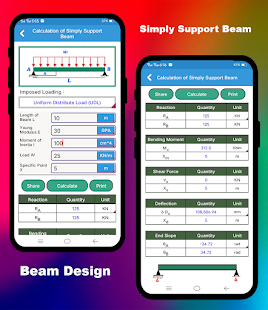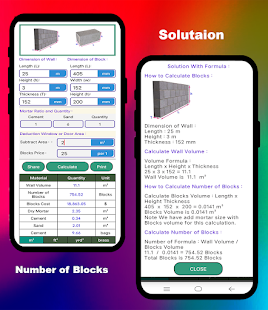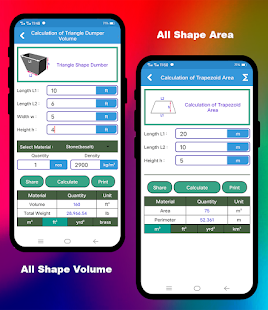Descriptions :

The civil calculation application is an Android application for the calculation of construction. In this application, we use a simple tool to simplify the calculation for the construction industry. The civil calculation app is a calculation platform for all civil engineers and construction workers, This civil calculator is useful for civil engineers, construction supervisor, civil engineering students, construction project manager, mechanical engineers, manager of construction shop, new engineers, construction contractor, construction contractor, store manager, site excitation engineer, quantity engineers (QS) and many more.

Faetures:
- Arch Wall Brick Calculator
- Concrete block calculator
- Plaster quantity calculator
- Fill quantity calculator
- Excavation and fill quantity calculator
- Paint quantity calculator
- Slope fill quantity calculator
- Asphalt amount calculator
- Tile quantity calculator
- Terrazzo quantity calculator
- Floor brick quantity calculator
- Antitermite quantity calculator
- Water tank calculator (circular and rectangular)
- Concrete test calculator
- Form job calculator
- Foundation depth calculator

Required Android Versions : Jelly Bean [4.2–4.3.1] - KitKat [4.4–4.4.4] - Lollipop [5.0–5.0.2] - Marshmallow [6.0 - 6.0.1] - Nougat [7.0 – 7.1.1] - Oreo [8.0-8.1]